# Using a shader to fade out GameObjects when they come close

Would you like to fade in or fade out objects in Unity based on their poximity to the camera? After reading this, you are able to create a Unity Shader that manipulates the alpha value of an objects material color based on a distance parameter.

• Create a new Unity project
• Create a cube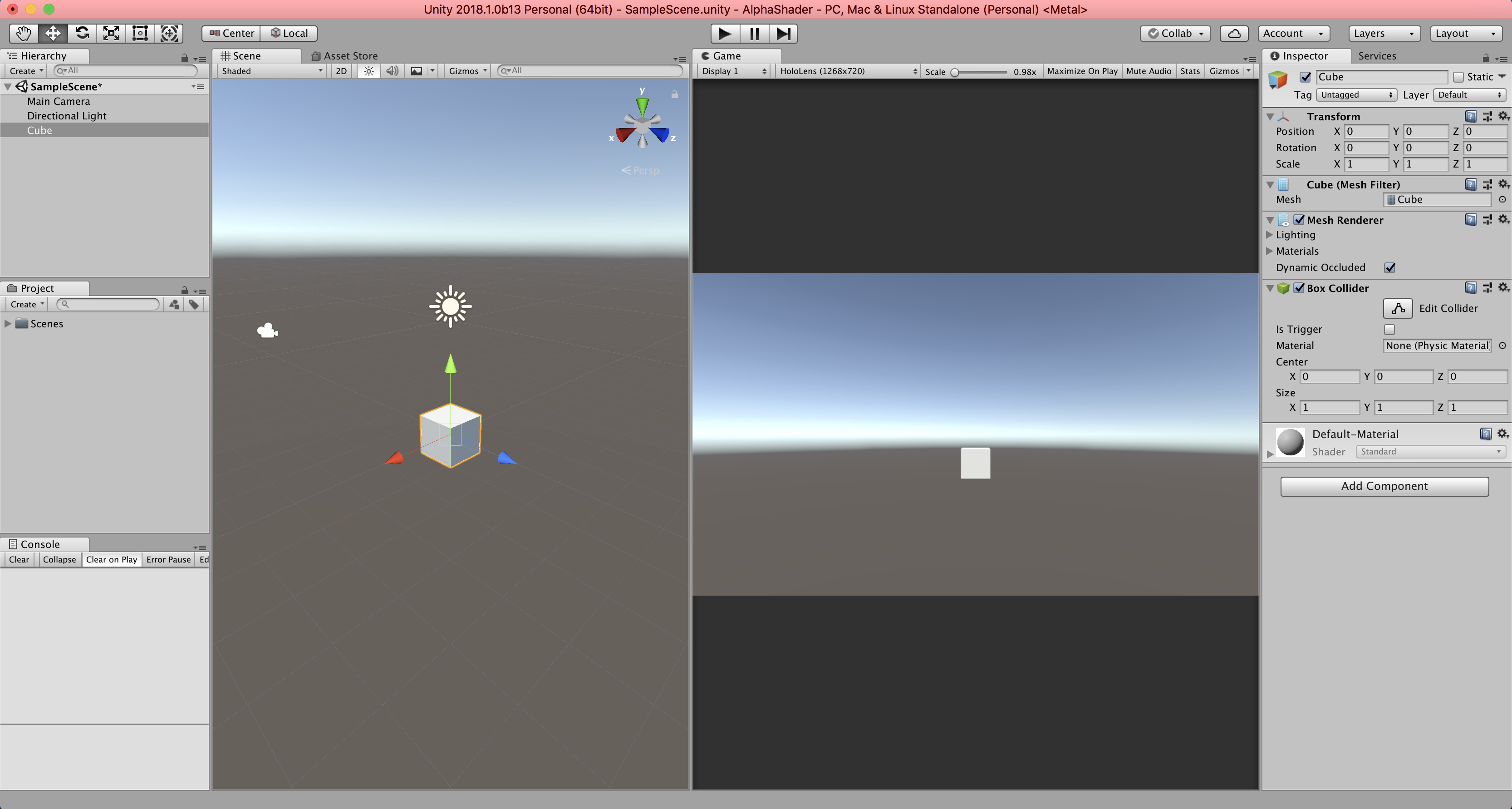By moving the camera positions `Z` value, the cube appears to come closer and as you would expect, the alpha value remains the same. In other words, the cube remains opaque.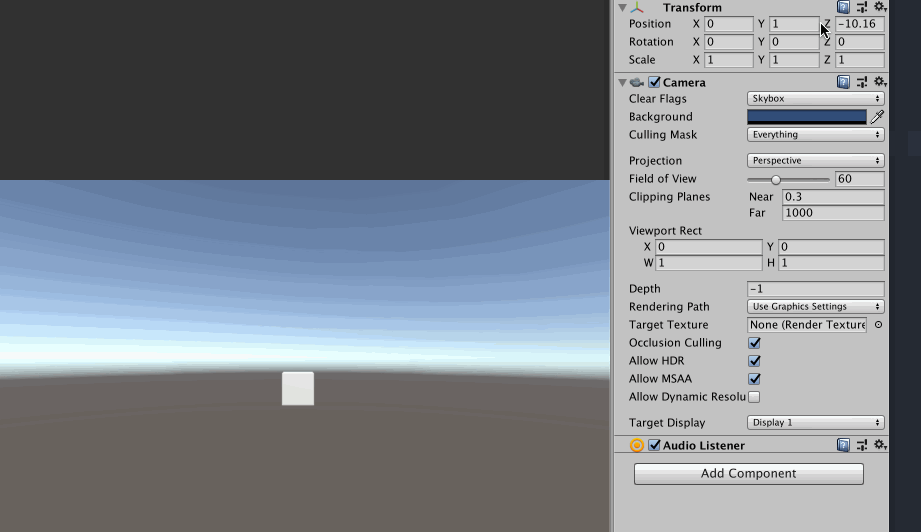## Create a shader that changes the Alpha value (opacity)

• Create an Image Effect Shader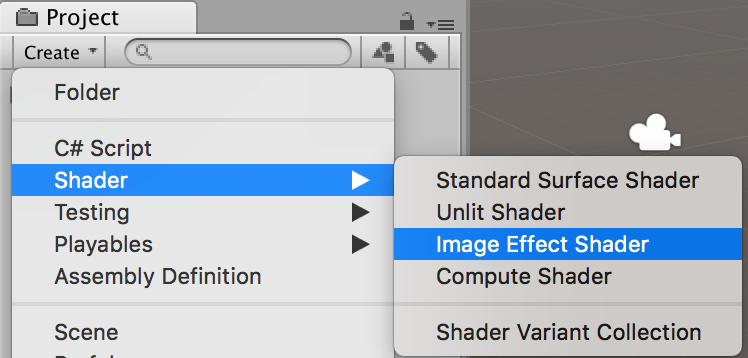• Name it `AlphaShader`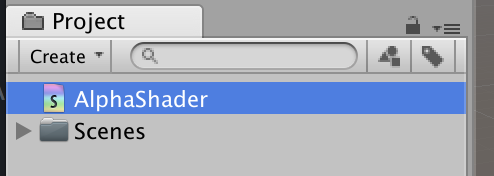• Doubleclick `AlphaShader` to open in Visual Studio
• Replace the `AlphaShader` code with:
``````Shader "AlphaShader"
{
{
Pass
{
CGPROGRAM
#pragma vertex vert
#pragma fragment frag

#include "UnityCG.cginc"

struct appdata
{
float4 vertex : POSITION;
float2 uv : TEXCOORD0;
};

struct v2f
{
float2 uv : TEXCOORD0;
float4 vertex : SV_POSITION;
};

v2f vert (appdata v)
{
v2f o;
o.vertex = UnityObjectToClipPos(v.vertex);
o.uv = v.uv;
return o;
}

fixed4 frag (v2f i) : SV_Target
{
return fixed4(i.uv.r, 1, 1, 1);
}
ENDCG
}
}
}
``````
• Create Material `AlphaMaterial`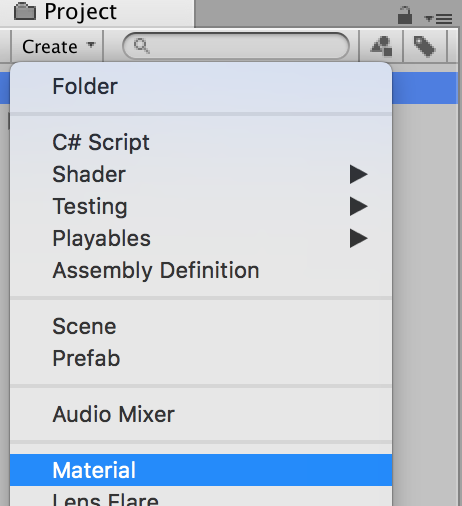• Drag `AlphaShader` on `AlphaMaterial`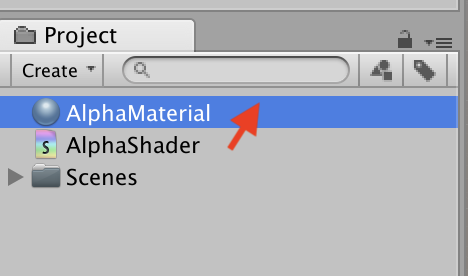• Drag `AlphaMaterial` on `Cube`As you see, the shader has been applied on the cube. Notice that the shader has this line,

``````return fixed4(i.uv.r, 1, 1, 1);
``````

which changes the red value (from 0 to 1) based on the horizontal pixel position, expressed in `uv.r` and creates a gradient effect. This is just to show you that the shader actually works. Since we don’t want a gradient, we are going to return a solid white color:

• Change the frag function:
``````fixed4 frag (v2f i) : SV_Target
{
return fixed4(1, 1, 1, 1);
}
``````

This will result in a white cube on the screen. Now let’s make it interactive!

Your `AlphaShader` should change its alpha value based on the distance to another object (for example the camera). It also needs to know when to start fading and when it needs to be faded out. So we need 3 properties:

1. Distance
3. FadeCompleteDistance (at this distance, the opacity is 0)

1. Declare Material Properties in a Property block
2. Declare Cg/HLSL variables (with the same name) in the shader code
``````Shader "AlphaShader"
{
Properties
{
_Distance ("Distance", Float) = 0
}

{
.
.
``````
``````SubShader
{
Pass
{
CGPROGRAM
#pragma vertex vert
#pragma fragment frag

#include "UnityCG.cginc"

float _Distance;
.
.
``````

## Enable transparency

By default, a Unity shader does not support transparency and the Color alpha value will be ignored. To enable transparency, add one more line to the shader:

``````SubShader
{
Pass
{
.
.
``````

Inspect your cube to see the new Material properties:You can already test the shader by directly changing `Distance`, `FadeStartDistance` and `_FadeCompleteDistance`. But how do we update the `Distance` at runtime?

## Using SetFloat to update the Shader Properties at runtime

• Select `Cube`
• Add Script `Proximity`
• Open the script in Visual Studio
• Replace the script code with:
``````using UnityEngine;

public class Proximity : MonoBehaviour
{
private Material _material;

private void Start()
{
_material = GetComponent<MeshRenderer>().material;
}

private void Update()
{
var distance = Mathf.Abs(Camera.main.transform.position.z - transform.position.z);
_material.SetFloat("_Distance", distance);
}
}
``````
• Switch back to Unity
• Press Play
• Select the camera
• Move the cameras `z` valueThe cube now fades in and out depending on the distance to the camera. For your convenience, here’s the full shader code:

``````Shader "AlphaShader"
{
Properties
{
_Distance ("Distance", Float) = 0
}

{
Pass
{

CGPROGRAM
#pragma vertex vert
#pragma fragment frag

#include "UnityCG.cginc"

float _Distance;

struct appdata
{
float4 vertex : POSITION;
float2 uv : TEXCOORD0;
};

struct v2f
{
float2 uv : TEXCOORD0;
float4 vertex : SV_POSITION;
};

v2f vert (appdata v)
{
v2f o;
o.vertex = UnityObjectToClipPos(v.vertex);
o.uv = v.uv;
return o;
}

fixed4 frag (v2f i) : SV_Target
{
return fixed4(1, 1, 1, 1); // no change
}